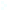Election CitationResearch Interests Name Brezis, Haim Location Rutgers, The State University of New Jersey, New Brunswick Primary Field Mathematics Secondary Field Applied Mathematical Sciences A mathematician from France, Brezis is an outstanding figure in nonlinear functional analysis and one of the world's principal leaders in the study of nonlinear partial differential equations. He has made path-breaking contributions to the application of mathematics to physical problems and geometry. My mathematical research has been devoted to the study of solutions of nonlinear partial differential equations and to a variety of problems in mathematical physics and differential geometry, involving such equations. In the past decade, my students and I have investigated models arising in the Thomas-Fermi theory of atoms and molecules, in the theory of liquid crystals and in superconductors. This includes a detailed mathematical analysis of singularities: line and point defects, Ginzburg-Landau vortices, etc. In the closely related problems of global geometry, blow-up phenomena and the appearance of singularities are associated with critical exponents and lack of compactness in the variational formulation. I have been able to classify all possible singular behavior in many cases, using new techniques and ideas from various sectors of mathematics: calculus of variations, nonlinear functional analysis, estimates in Sobolev spaces of solutions of nonlinear partial differential equations, Fourier analysis and the topology of Sobolev manifolds.